The ROBUSTREG Procedure
 Robust Distance

The ROBUSTREG procedure uses the robust multivariate location and scale estimates for leverage-point detection. The procedure computes a robust version of the Mahalanobis distance by using the minimum covariance determinant (MCD) method of Rousseeuw (1984).

### Algorithm

PROC ROBUSTREG implements the algorithm given by Rousseeuw and Van Driessen (1999) for MCD, which is similar to the algorithm for LTS.

### Robust Distance

The Mahalanobis distance is defined as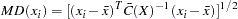where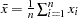and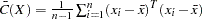are the empirical multivariate location and scale. Here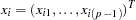does not include the intercept. The relation between the Mahalanobis distance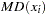and the hat matrix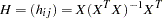is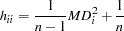The robust distance is defined as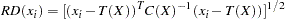where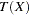and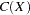are the robust multivariate location and scale obtained by MCD.

These distances are used to detect leverage points.Previous Page | Next Page | Top of Page